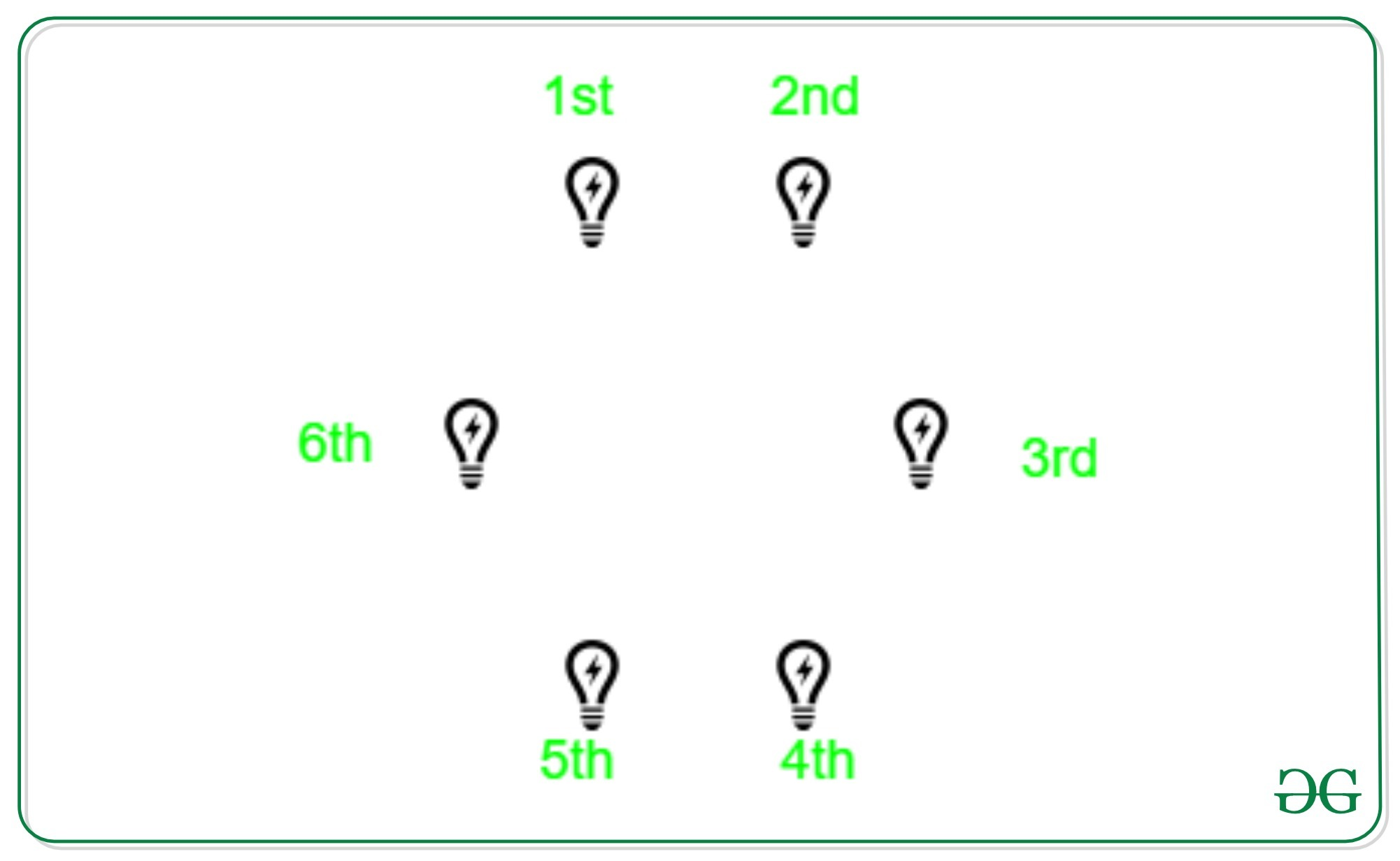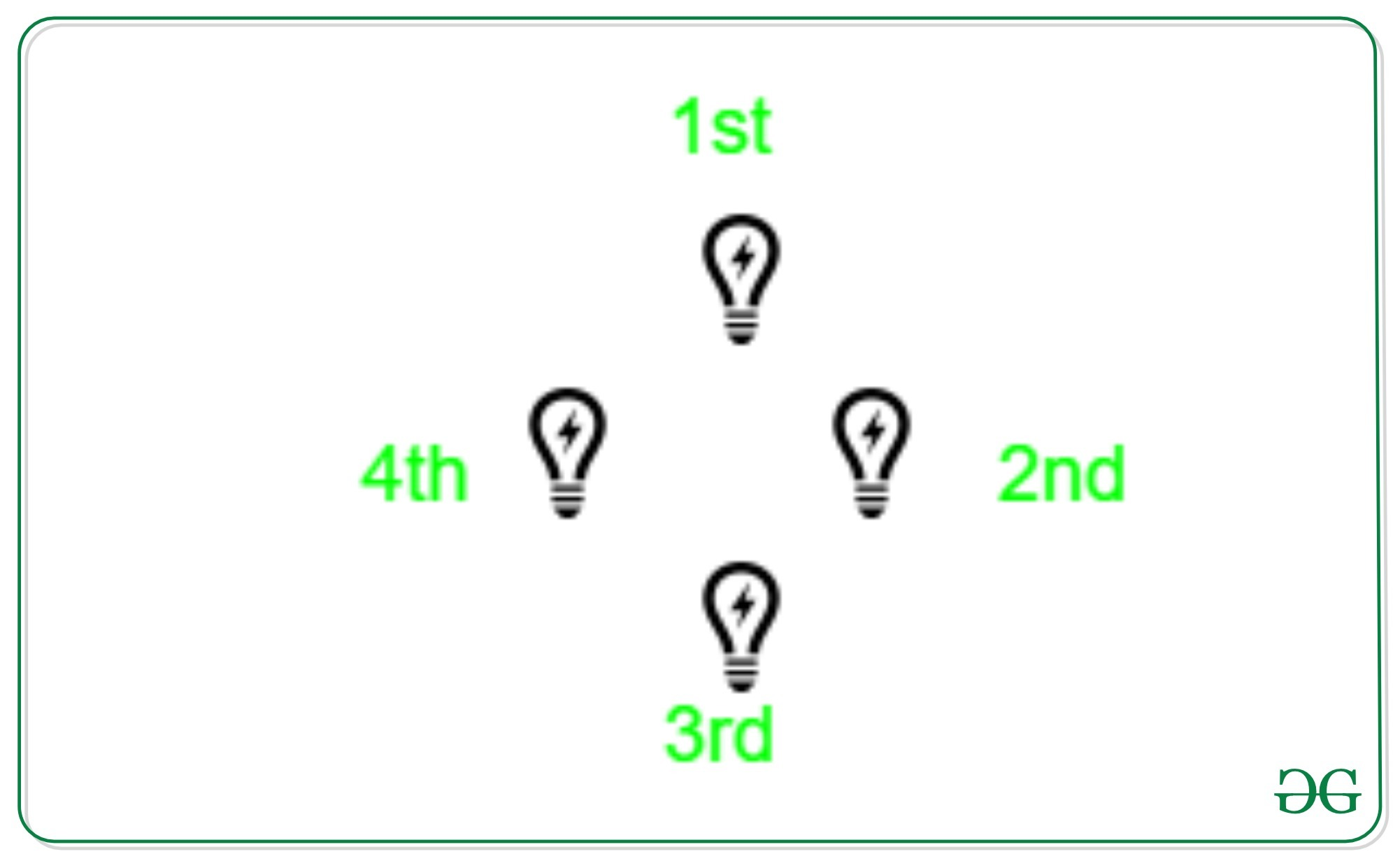Skip to content
Related Articles
Puzzle | The Circle of Lights
• Difficulty Level : Hard
• Last Updated : 01 Jul, 2020

There is a circle of N > 2 lights with a switch next to each of them. Each switch can be flipped between two positions, thereby toggling the on/off states of three lights: its own and the two lights adjacent to it. Initially, all the lights are off. Design an algorithm for turning all the lights on by flipping the minimum number of switches.

Solution:
Since all the lights are off initially, it is obvious that the final state of the lights depends only on the parity(odd or even) of the number of times each switch is flipped and does not depend on the order in which the switches are manipulated. To turn on a light, we can either flip its switch and leave the adjacent switches off, or we flip all the three switches, for a group of three lights. Assuming the lights are numbered from 1 to N in the clockwise order, we have two cases.

1. N is divisible in 3: In this case, we flip the switch for 1st bulb, which lights the bulbs 1, 2 and N. Then we flip the switch of 4th bulb, which lights the bulb 3, 4, and 5. In this manner, we flip the switch of every 3K + 1 bulb until we flip the switch of N – 2 bulb, which will light up the last 3 bulbs. This results in a total of N/3 switch flips.2. N is not divisible by 3: In this case, suppose we follow the same procedure as above. This will only turn on the bulbs in multiple of 3. So at last, there will be one or two bulbs, which will be off. Flipping the switch of one of these bulbs, we turn off at least one bulb. This bulb, say X, which now has gone off due to the previous flip has been toggled even number of times, to turn it on, the switch of either X or one of its adjacent bulbs which hasn’t been flipped needs to be flipped. In this way, we have to flip the switch of each of the bulb whose switch hasn’t been flipped. This results in a total of N switch flips.My Personal Notes arrow_drop_up